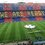# Circles

Given that $\omega_1$ and $\omega_2$ are two circles which intersects at points $X$ and $Y$. Let $P$ be an arbitrary points on $\omega_1$. Suppose that the lines $PX$ and $PY$ meet $\omega_2$ again at points $A$ and $B$, respectively.

Prove that the circumcircles of all triangles $PAB$ have the same radius.Note by Sayantan Saha
4 years, 9 months ago

This discussion board is a place to discuss our Daily Challenges and the math and science related to those challenges. Explanations are more than just a solution — they should explain the steps and thinking strategies that you used to obtain the solution. Comments should further the discussion of math and science.

When posting on Brilliant:

• Use the emojis to react to an explanation, whether you're congratulating a job well done , or just really confused .
• Ask specific questions about the challenge or the steps in somebody's explanation. Well-posed questions can add a lot to the discussion, but posting "I don't understand!" doesn't help anyone.
• Try to contribute something new to the discussion, whether it is an extension, generalization or other idea related to the challenge.

MarkdownAppears as
*italics* or _italics_ italics
**bold** or __bold__ bold
- bulleted- list
• bulleted
• list
1. numbered2. list
1. numbered
2. list
Note: you must add a full line of space before and after lists for them to show up correctly
paragraph 1paragraph 2

paragraph 1

paragraph 2

[example link](https://brilliant.org)example link
> This is a quote
This is a quote
    # I indented these lines
# 4 spaces, and now they show
# up as a code block.

print "hello world"
# I indented these lines
# 4 spaces, and now they show
# up as a code block.

print "hello world"
MathAppears as
Remember to wrap math in $$ ... $$ or $ ... $ to ensure proper formatting.
2 \times 3 $2 \times 3$
2^{34} $2^{34}$
a_{i-1} $a_{i-1}$
\frac{2}{3} $\frac{2}{3}$
\sqrt{2} $\sqrt{2}$
\sum_{i=1}^3 $\sum_{i=1}^3$
\sin \theta $\sin \theta$
\boxed{123} $\boxed{123}$

Sort by:

I have sent IMO books and KVS RMO 2016 paper to your e-mails.

- 4 years, 8 months ago

Thanks a lot bro.

- 4 years, 8 months ago

I have 36 Olympiad books related to IMO. Do you want all of them?

- 4 years, 9 months ago

Yeah I also want em all.

- 4 years, 9 months ago

yup.sure.send it to my email.

- 4 years, 9 months ago

Ok no problem.

Can you tell me the author of co-exter book?

- 4 years, 9 months ago

H. S. M. Coxeter and S. L. Greitzer

- 4 years, 9 months ago

Is it Geometry revisited by MAA?

- 4 years, 9 months ago

yup

- 4 years, 9 months ago

That is not so good. It's good for reading only. It has less computational problems.

- 4 years, 9 months ago

yup.i use it to learn new stuff.

- 4 years, 9 months ago

Do you have any good book regarding NUMBER THEORY?

- 4 years, 9 months ago

can u give ur email ID

- 4 years, 9 months ago

I gave. See above.

- 4 years, 9 months ago

David M.burton book;s

- 4 years, 9 months agoProblem 1

We can see that as we move the point $P$ on the circumference of the circle$[$excluding $X$ and $Y],$the $\angle XPY=\angle XP_1Y$ remains constant.So this shows that $AB=A_1B_1.$Now we use extended sin rule to complete the problem.
Let the circum-radius of $\triangle PAB$ be $R$ and $\triangle P_1A_1B_1$ be $R_1.$
In $\triangle PAB, \frac{AB}{sin\angle P}=2R$ and in $\triangle P_1A_1B_1,\frac{A_1B_1}{sin\angle P_1}=\frac{AB}{sin\angle P}=2R_1.$ Therefore $2R=2R_1\Rightarrow\boxed {R=R_1}.$Hence Proved.

- 4 years, 9 months ago

Very good solution. Very much clear also. I understood it now.

- 4 years, 9 months ago

Thanks...My fav. is geometry.I see that u like olympiad problems.Can u give some links of ur problems?did u get selected in INMO??

- 4 years, 9 months ago

I have many interesting books regarding IMO. I post problems from there only.

I have that books in PDF.

Actually I am attending KVS INMO camp for INMO 2017. I have to give INMO directly.

By the way, from which book you do geometry problems?

- 4 years, 9 months ago

Please share these resources with me also.

My email id : harshus99@gmail.com

- 4 years, 9 months ago

and also give the INMO paper to this email.Did u write RMO this year

- 4 years, 9 months ago

Yes I appeared in RMO this year from KVS region.

- 4 years, 9 months ago

How many did u solve?

- 4 years, 9 months ago

I solved 4 and half.

- 4 years, 9 months ago

Awesome! Send me the question paper also then

- 4 years, 9 months ago

You will get it.

I am currently out of Delhi. I will send you after 5 Nov.

- 4 years, 9 months ago

ok.no probs. Can u give ur email address

- 4 years, 9 months ago

priyanshu_2feb@rediffmail.com

- 4 years, 9 months ago

Sometimes co-exter or pre-college maths.Can u give the pdf of the books to this email : raiayush234@gmail.com

- 4 years, 9 months ago

This is there in IF Sharygin, plane geometry.

- 4 years, 9 months ago

A problem copied from IF Sharygin Plane geometry. This is bad.

- 4 years, 9 months ago

Why will I copy? This is RMO Delhi's problem

- 4 years, 9 months ago

What I am saying and what are you understanding.

I am blaming RMO people that they have took this problem from a popular book which usually does not happen.

- 4 years, 9 months ago

The inequality problem in Delhi region was also copied.I don't remember the source, but I had seen it before RMO on some forum.

This is disappointing :(

- 4 years, 9 months ago

Actually this year Delhi paper was prepared by Delhi coordinator only not by HBCSE. So he copied many things because framing a question requires a lot of time.

- 4 years, 9 months ago

rmo dehli right

- 4 years, 9 months ago

yeah.Are you appearing for this year's rmo?

- 4 years, 9 months ago